# The eight Thurston geometries

## From Euclid to Thurston… and beyond

### Euclid and the parallel postulate

300 years BC, Euclid summarizes in his work The Elements the geometric knowledge of his time. This book had a strong influence beacuse of his deductive treatment of mathematics. In particular Euclid states in the first book five postulates from which everything else should be deduced. While the first four postulate are very “natural” (e.g. given any two points, there is a segment joining them) the fifth postulate, also knwon as the parallel postulate plays a different role.

There are numerous equivalent forms of the fifth postulate. Here are a few of them.

• If a line segment intersects two straight lines forming two interior angles on the same side that are less than two right angles, then the two lines, if extended indefinitely, meet on that side on which the angles sum to less than two right angles.
• There is at most one line that can be drawn parallel to another given one through an external point.
• The sum of the angles in every triangle is $2\pi$ (i.e. 180°)

### The emergence of non-euclidean geometries

For a long time nobody doubt about the validity of the fifth postulate. Nevertheless, many mathematicians tried to deduce it from the first four… in vain. It was only during the 19th century that several mathematicians (Gauß, Bolyai, and Lobatchevski) independently realized that one can build “other geometries” where the first four postulate hold while the fifth one fails. This breakthrough demonstrates that the parallel postulate is not a consequence of other four. This is the beginning of non-euclidean geometry.

### Classifying geometric structures

Knowing that there exist several distinct geometries, a natural question is to classify all of them. In dimension two, the solution of this problem is given by the Uniformization Theorem. Roughly speaking, it states that any two-dimensional compact manifold can be endowed with a metric with constant curvature. If the curvature is positive (respectively zero, negative) then the geometry is modeled on the two-sphere (respectively the euclidean plane, the hyperbolic plane).

In dimension three, the situation is more complicated: a compact three-manifold does not always admit a geometric structure. However, Thurston’s conjecture, proved by Perelman, states that any compact three-manifold can be cut along essential spheres and tori so that the interior of each resulting piece admits a geometric structure. There are eight possible geometric structures, called Thurston’s geometries.

This website allows you to experiment what it would be to live in each of them.

## A first look at the Thurston geometries

Warning: The simulations below are working real-time on your computer. They include numerous objects and shading effects requiring a powerful graphic card. If your computer is not fast enough, you can reduce the size of your browser window. You can also explore each geometry page to try less greedy simulations.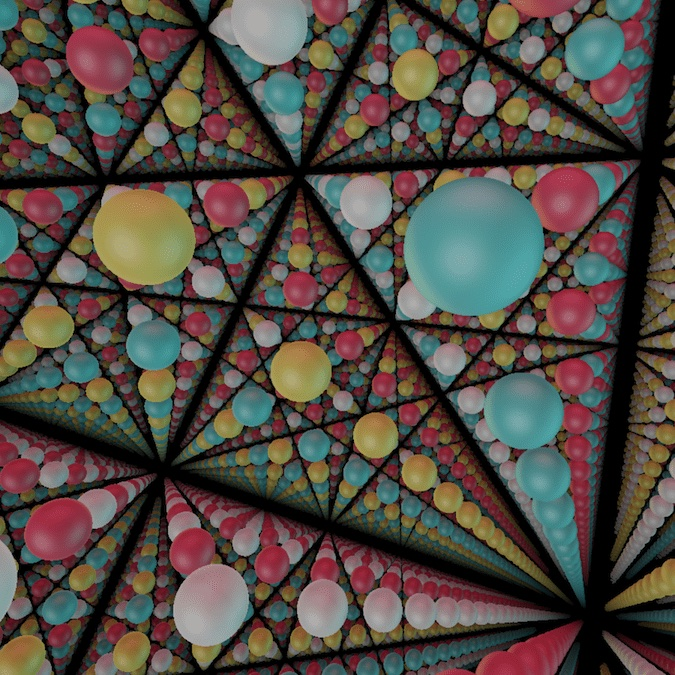##### Euclidean

A collection of eight spheres in the 3-torus.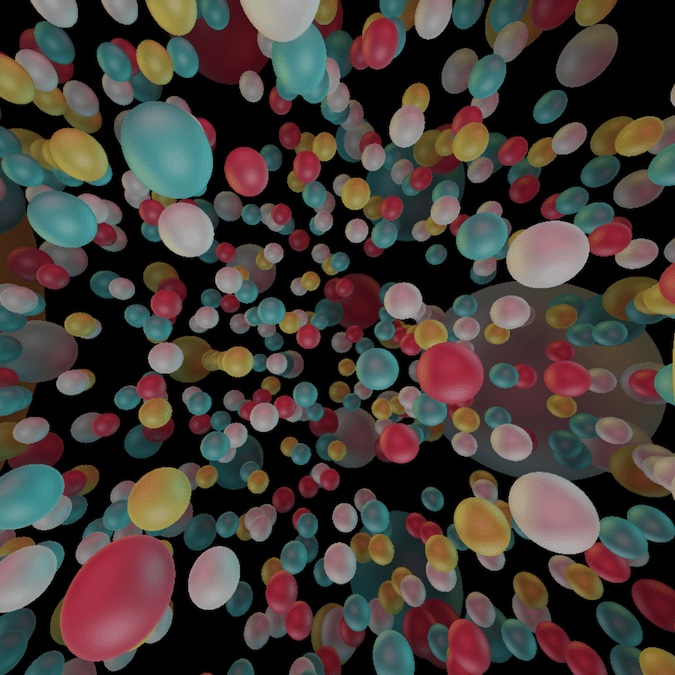##### Spherical

A collection of eight spheres in the 3-torus.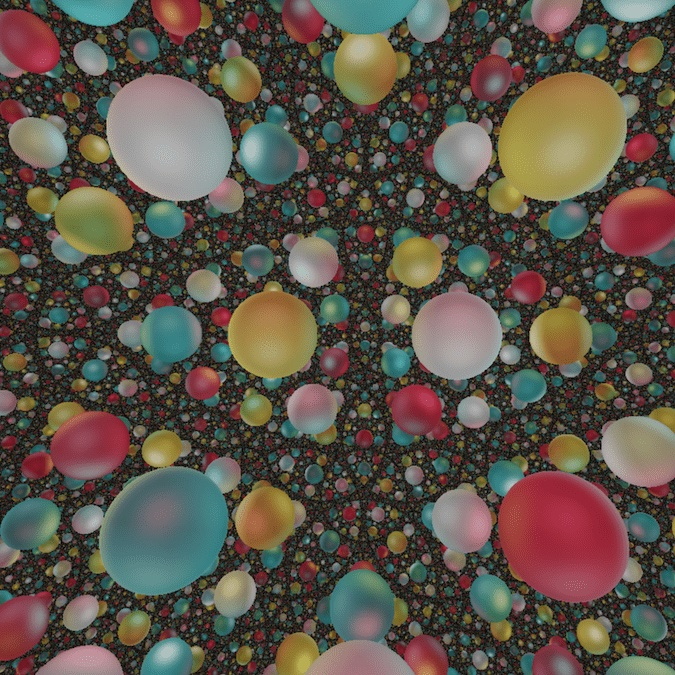##### Hyperbolic

A collection of twelve spheres in Seifert-Weber dodecahedral space.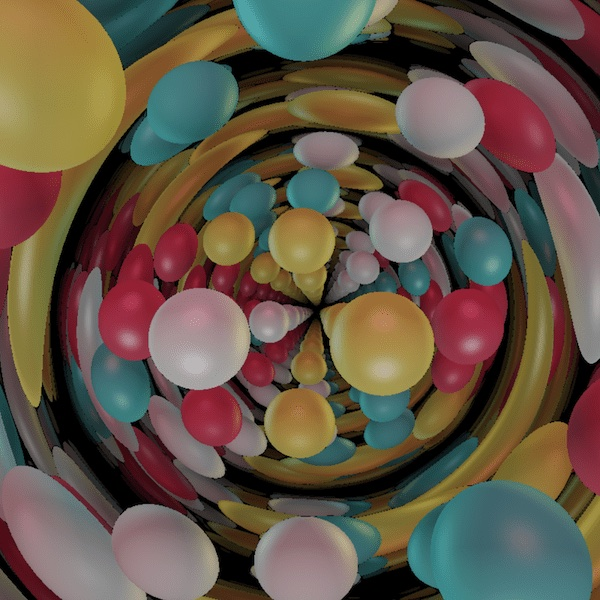##### $S^2 \times \mathbb E$

A collection of 20 spheres in the Hopf manifold $S^2 \times S^1$, arranged on the vertices of an icosahedron in the $S^2$ factor.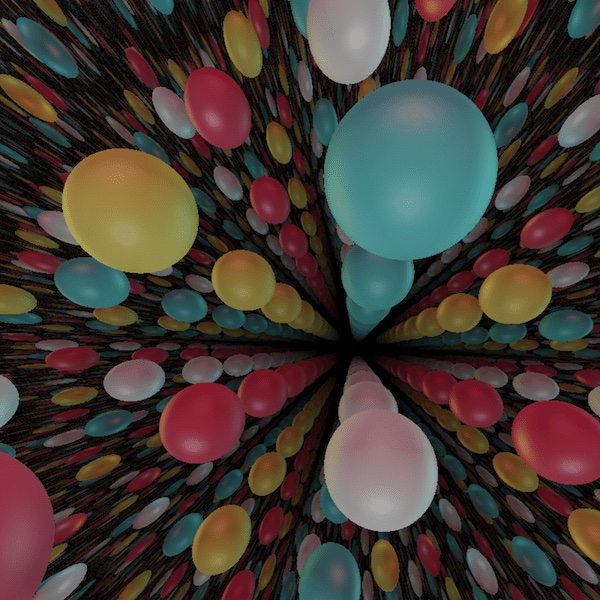##### $\mathbb H^2 \times \mathbb E$

A collection of four spheres in the trivial circle bundle over a hyperbolic orbifold torus with cone angle $\pi$.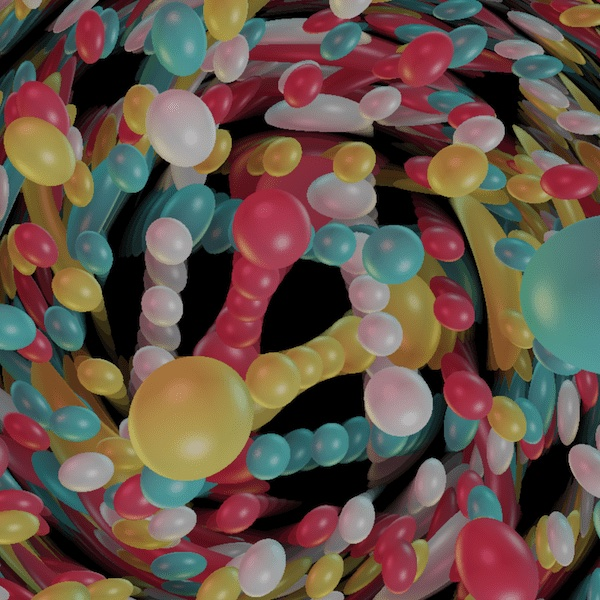##### Nil

A collection of four spheres in a Dehn twist torus bundle.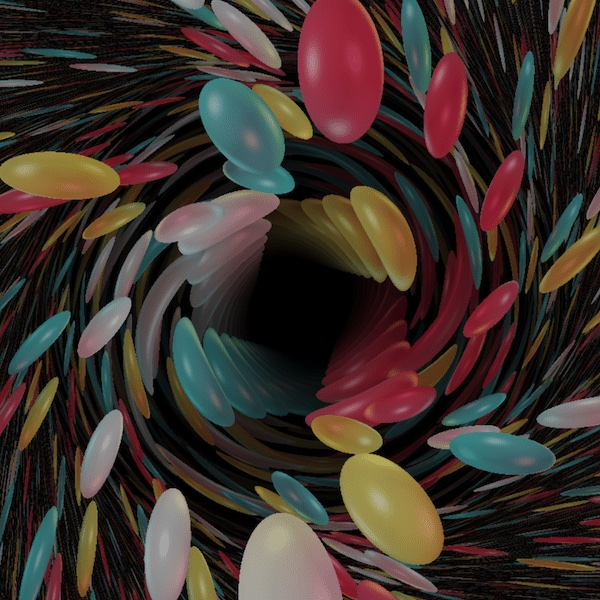##### $\widetilde{\rm SL}(2,\mathbb R)$

A collection of four spheres in the unit tangent bundle to a hyperbolic orbifold torus with cone angle $\pi$.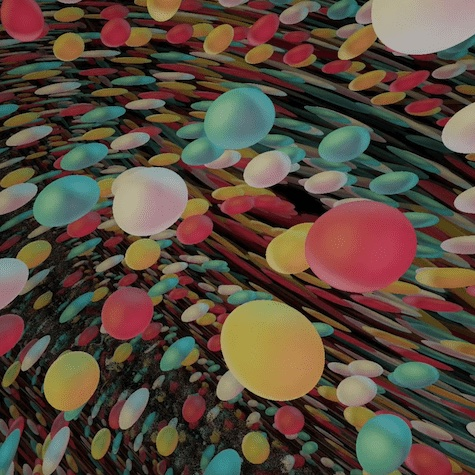##### Sol

A collection of four spheres in an Anosov torus bundle.

## The geometries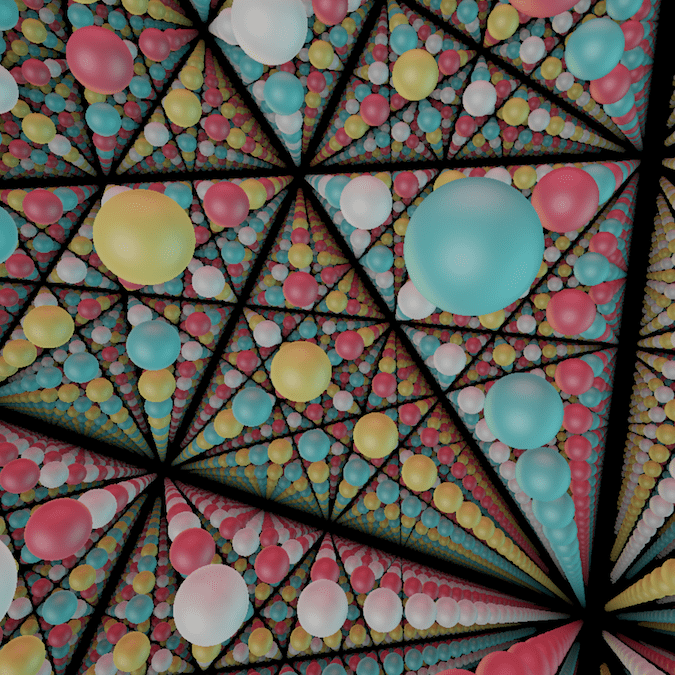### The euclidean space $\mathbb E^3$

The euclidean space corresponds to the “usual” 3D geometry we learn at school. It is the geometry of the flat real 3-dimensional vector space $\mathbb R^3$.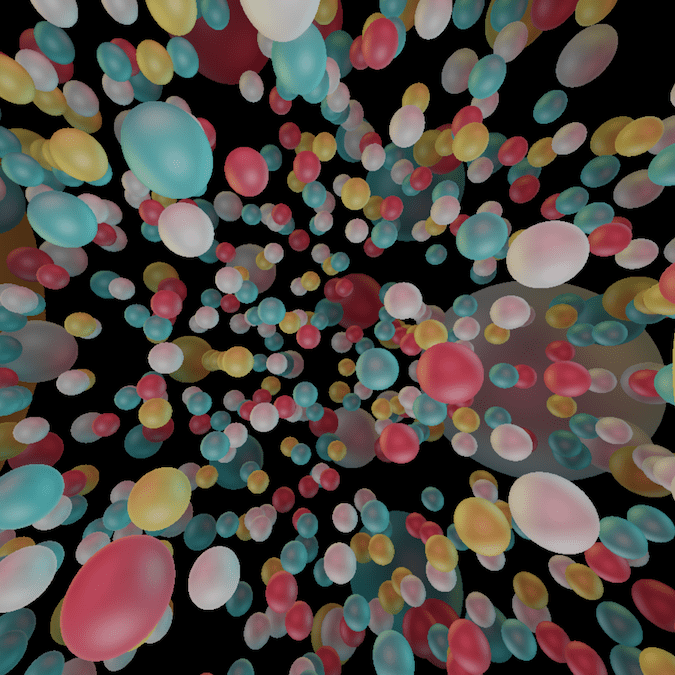### The spherical space $S^3$

The space $S^3$ is the 3-dimensional analogue of the usual sphere in 2 dimensions. It is an isotropic space (all the directions play the same role). Among the eight Thurston geometries, it is the only compact one. It can be seen as the unit tangent bundle of the 2-sphere. This point of view is also knwon as the Hopf fibration.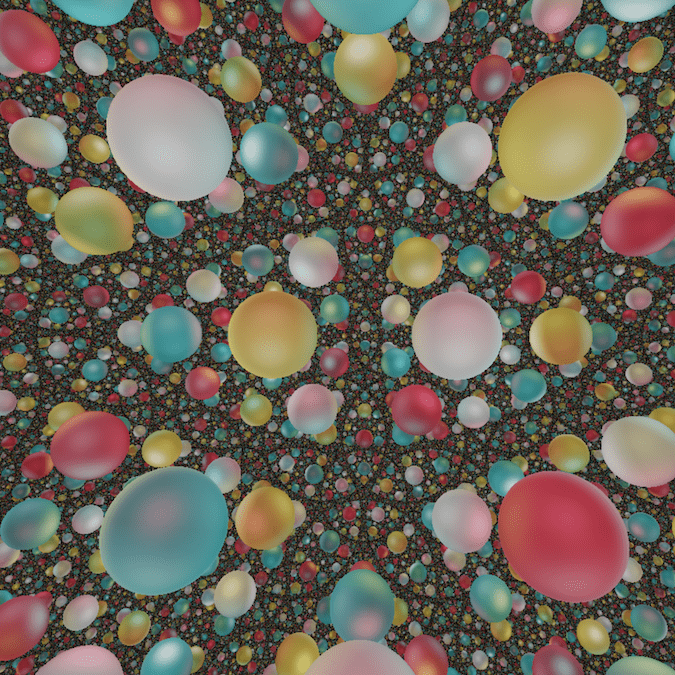### The hyperbolic space $\mathbb H^3$

The hyperbolic space $\mathbb H^3$ is the three-dimensional analog of the hyperbolic plane. It is an isotropic space (all the directions play the same role). Among the eight geometries, this is probably the one which has the richest class of lattices.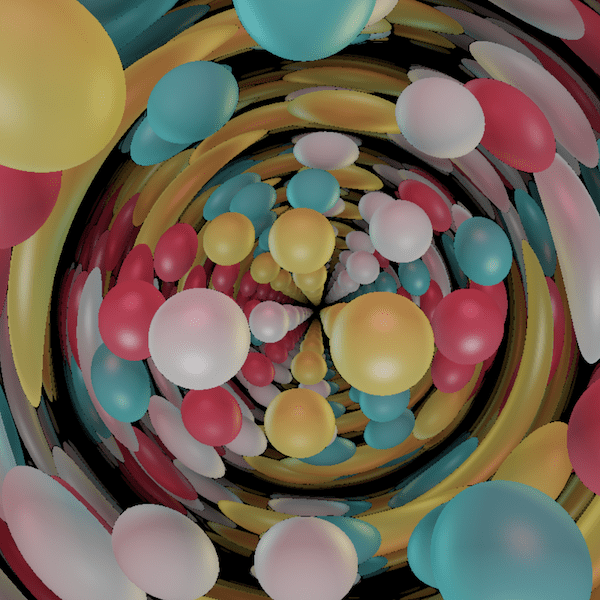### The product geometry $S^2 \times \mathbb E$

This geometry is the cartesian product of the two-sphere and the real line. It can be also seen as the universal cover of the product $M = S^2 \times S^1$ where $S^2$ is the two-sphere and $S^1$ the unit circle.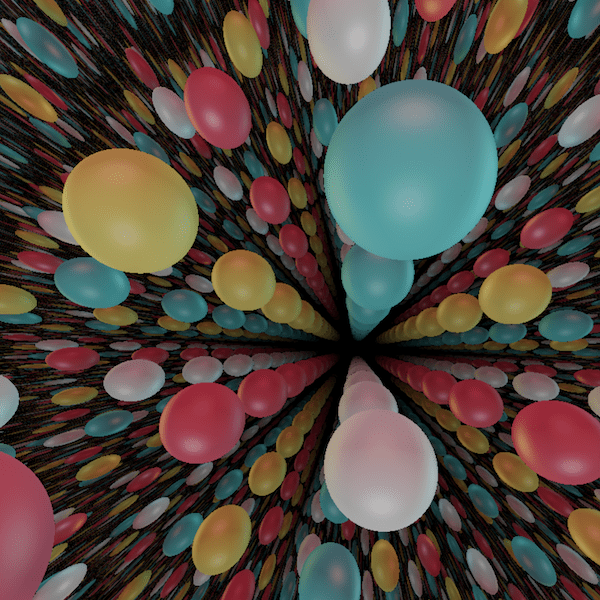### The product geometry $\mathbb H^2 \times \mathbb E$

This geometry is the cartesian product of the hyperbolic plane and the real line. It can be also seen as the universal cover of the product $M = \Sigma_g \times S^1$ where $\Sigma_g$ is a compact surface of genus $g \geq 2$ and $S^1$ the unit circle.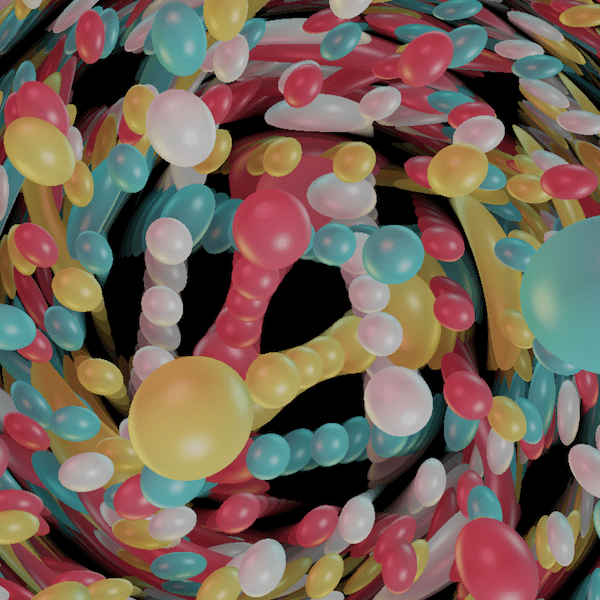### The Nil geometry

Nil is a 3-dimensional nilpotent Lie group. It can also be seen as the universal cover of the suspension of a 2-torus by a Dehn twist.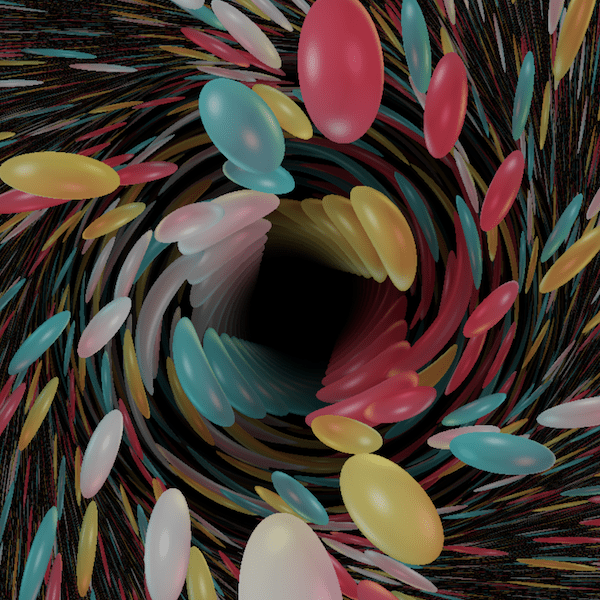### The universal cover of ${\rm SL}(2,\mathbb R)$

${\rm SL}(2,\mathbb R)$ is the set of all $2 \times 2$-real matrices with determinant one. The space $\widetilde{\rm SL}(2,\mathbb R)$ is its universal cover. There are several ways to think about this space. ${\rm SL}(2,\mathbb R)$ is for instance the unit tangent bundle of the hyperbolic plane $\mathbb H^2$. This point of view gives $\widetilde{\rm SL}(2,\mathbb R)$ a structure of a twisted metric line bundle over $\mathbb H^2$, which can be thought as a hyperbolic analogue of the Hopf fibration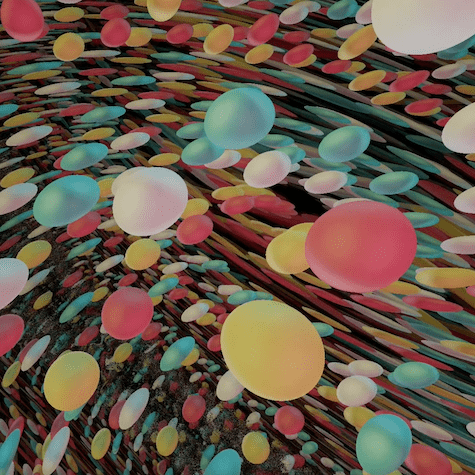### The Sol geometry

Sol is a 3-dimensional solvable Lie group. It can also be seen as the universal cover of the suspension of a 2-torus by an Anosov matrix.